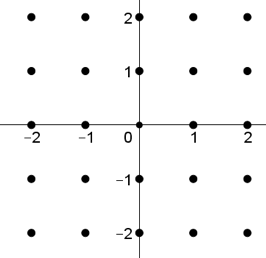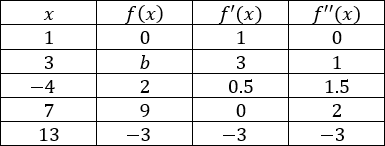# AP Calculus AB — Practice Exam Free Response Part B

Here is Part B of our free AP Calculus AB free response questions. This is the second FRQ section of our AP Calculus AB Practice Exam. After you write down your solutions you can check your answers here. A graphing calculator is not allowed for these problems.

Question #3

Consider the differential equation $\dfrac{dy}{dx} = x^3 − 2y^2$.

(a) Sketch the slope field for the differential equation on the graph provided below.(b) Find $\dfrac{d^2y}{dx^2}$ in terms of $x$ and $y$. Determine the concavity of the possible solution curves to the differential equation in the second quadrant based on your answer.

(c) Given the differential equation $\dfrac{dy}{dx} = 2x^3y^2$, solve for $y$ in terms of $x$.

Question #4

Tim and John get in an argument over the limit below.

$\lim\limits_{x\to 0} ⁡\dfrac{2x}{\cos(x)}$

Tim says the limit exists while John says the limit does not exist.

(a) Identify derivative of $x^2$ using the limit definition.

(b) Identify the derivative of $\sin⁡(x)$ using the limit definition.

(c) Identify $\lim\limits_{x\to 0} ⁡\dfrac{2x}{\sin(x)}$

Question #5

A particle moves along the $x$-axis over time, $t$, in seconds by the equation below. $t ≥ 0$

$s(t) = t^3 − 6t^2 + 9t − 18$

(a) Find the velocity and acceleration functions for the particle.

(b) When is the particle at rest?

(c) When is the particle moving in the forward direction?

(d) When is the particle increasing in speed?

(e) When is the particle moving the fastest in the backwards direction?

(f) Find the total distance travelled by the particle after $5$ seconds.

Question #6

Use the table below to answer all parts of the question. Assume that $f(x)$ is continuous and differentiable on all $x$. $f'(x) = 0$ only at one value for all $x$.(a) Identify the critical point(s) and point(s) of inflection for $f(x)$. Give a reason for each answer.

(b) Identify any local extrema for $f(x)$, stating reasons for each extremum identified.

(c) Using a tangent line approximation from $f(1)$, identify an appropriate approximation for b.

(d) How many roots does $f(x)$ have on $−4 ≤ x ≤ 13$? State a reason for your answer.